Time: 3 Hours                                                                                                     Max. Marks: 100

NOTE: There are 9 Questions in all.

·      Question 1 is compulsory and carries 20 marks. Answer to Q. 1. must be written in the space provided for it in the answer book supplied and nowhere else.

·      Out of the remaining EIGHT Questions answer any FIVE Questions. Each question carries 16 marks.

·      Any required data not explicitly given, may be suitably assumed and stated.

Q.1       Choose the correct or best alternative in the following:                                         (2x10)

a.       A common standard for serial communication is

(A)  ASCII.                                         (B)  EBCDIC.

(C)    RS-232.                                      (D)  Centronics.

b.      The main purpose of computer network is

(A)    Resource sharing.                         (B)  Computation speed up.

(C)  Reliability.                                    (D)  Communication.

c.   In the ________ layer, the data unit is called a packet.

(A)    Physical layer.                              (B)  Data link layer.

(C)  Transport layer.                            (D)  Network layer.

d.   In HDLC, ABM means

(A)    Automatic Balanced Mode.         (B)  Asynchronous Balanced Mode.

(C)  Assymetrical Balanced Mode.      (D)  Automatic Back-up Mode.

e.   For stop and wait ARQ protocol, the maximum utilisation of the link is given by ______where parameters given have usual significance

(A)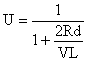(B)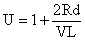(C)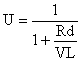(D)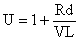f.    The __________ houses the switches in Token Ring.

(A)     NIC                                             (B)  MAU

(C)  nine-pin connector                        (D)  Transceiver

g.   BISDN is an acronym for

(A)     Broadband Information Services for Digital Networks

(B)     Broadband Internetwork System for Data Networks

(C)     Broadband Integrated Services Digital Network

h.   Which of the following operates in all seven layers of the OSI model

(A)    Bridge                                          (B) Gateway

(C) Routers                                         (D) All of the above

i.    The data unit in the TCP layer is called a ___________

(A)   Message                                       (B) Segment

(C) Datagram                                      (D) Frame

j.    The X.25 protocol operates in the _________ of the OSI model

(A)  Physical layer                               (B)  Data link layer

(C)  Network layer                              (D)  Transport Layer

Answer any FIVE Questions out of EIGHT Questions.

Each question carries 16 marks.

Q.2     a.   Discuss the principles used in defining the OSI layers.                                         (8)

b.   What is flow control in computer networks? Explain.                                          (8)

Q.3     a.   A telephone line normally has a bandwidth of range 300 Hz to 3300 Hz.  The signal to noise ratio is usually 3162 (35 dB).  For this channel, calculate the capacity in kbps.                                          (6)

b.   Explain the importance of DCE, DTE as applied to data communication.             (5)

c.   Assume that you are to design a TDM carrier, say DS-489, to support 30 voice channels using 6 bit samples and a structure similar to DS-1. Determine the required bit rate.                                          (5)

Q.4     a.   How network access layer is involved in TCP/IP layers.                                     (6)

b.   Explain the key services provided by the transport layer in detail.                      (10)

Q.5     a.   How do the layer of the TCP/IP protocol suite correlate to the layers of the OSI model?                (10)

b.   Discuss the ARQ protocols you have studied and compare them.                        (6)

Q.6     a.   Explain the elements required for packet switching networks.                              (8)

b.   List at least five different strategies for controlling congestion.                              (8)

Q.7     a.   Can bridging be used along with routers?  Explain.                                              (8)

b.   Differentiate between

(i)                  Twisted Pair and Co-axial cable.

(ii)                Network layer and Data Link Layer.                                   (4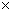2)

Q.8           Write short notes on the following:

(i) HDLC   (ii) Wireless networks   (iii) IPV6         (iii) ATM cells                 (44)

Q.9     a.   Explain the specifications in detail, for the following protocols.                             (6)

(i) FTAM  (ii) HTTP (III) SNMP

b.   Give the concept of electronic mail operation.                                                     (4)

c.   Discuss frame relay congestion control techniques.                                              (6)